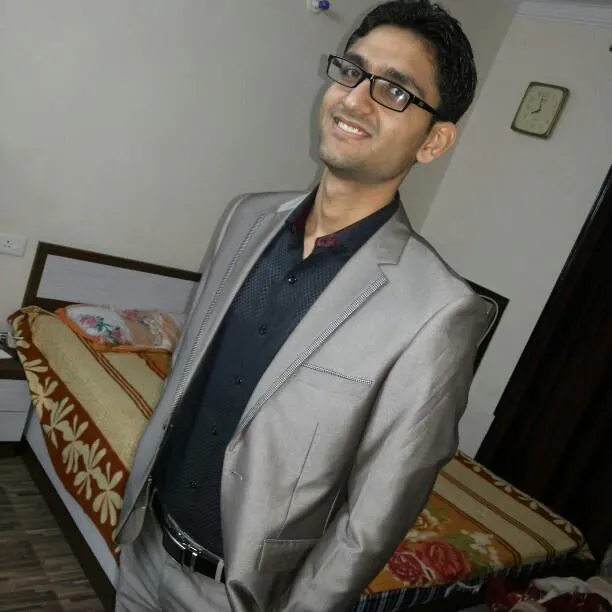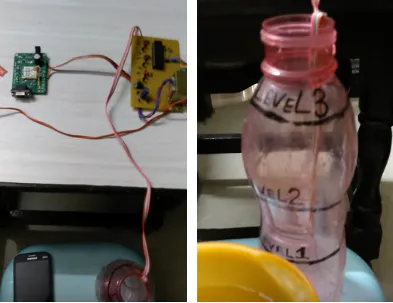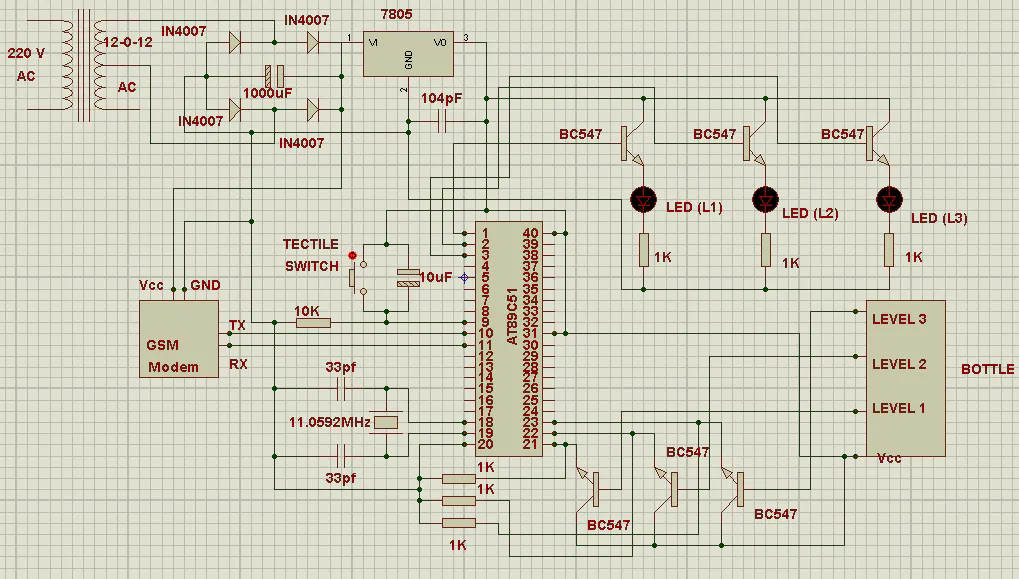# Water level indicator with SMS alert for emergency flood

whenever the level of water ir raised from a particular level it is not indicated to far away people for that this project is designed

IntermediateShowcase (no instructions)4,080## Things used in this project

### Hardware components

 transformer 12 - 0 - 12
×1
 connecting wire
×1
 2 pin power supply connector
×1
 GSM modem
×15 mm LED: Red
×3Resistor 10k ohm
×1
 at89c51 ic
×1
 40 pin ic base
×1
 bc547 transistor
×1
 crystal 11.0592mhz
×1
 33 pf
×1
 1000uf
×1
 10 uf
×1

### Hand tools and fabrication machinesSoldering iron (generic)
 soldering wire

## Schematics

### diagram## Code

### water_level_gsm.c

C/C++
```#include<reg51.h>

sbit level_1=P2^0;
sbit level_2=P2^1;
sbit level_3=P2^2;

sbit level_indication_1=P1^0;
sbit level_indication_2=P1^1;
sbit level_indication_3=P1^2;

void delay(unsigned int x)
{
unsigned int i;
for(i=0;i<=x;i++);
}

void tx(unsigned char num)
{
SBUF = num;
while(TI == 0);
TI = 0;
delay(500);
}

void tx_string(unsigned char *y)
{
while(*y!='\0')
{
tx(*y);
y++;
delay(500);
}
}
void main()
{
unsigned char a,b,c;
level_indication_1=0;
level_indication_2=0;
level_indication_3=0;
SCON=0x50;
TMOD=0x20;
TH1=0xfd;
TR1=1;

tx_string("AT");
tx(0x0d);
delay(60000);
tx_string("AT+CMGF=1");
tx(0x0d);
delay(60000);

while(1)
{

if(level_1==1)
{
if(a==0)
{
level_indication_1=1;
a=1;
tx_string("AT+CMGS=");
tx('"');
tx_string("09464519438");
tx('"');
tx(0x0d);
delay(60000);
tx_string("LEVEL 1 REACHED");
tx(0x1a);
delay(60000);
delay(60000);
delay(60000);
}
}
else
{
level_indication_1=0;

a=0;
}
if(level_2==1)
{
if(b==0)
{
level_indication_2=1;
b=1;
tx_string("AT+CMGS=");
tx('"');
tx_string("09464519438");
tx('"');
tx(0x0d);
delay(60000);
tx_string("LEVEL 2 REACHED");
tx(0x1a);
delay(60000);
delay(60000);
delay(60000);
}
}
else
{
level_indication_2=0;
b=0;
}
if(level_3==1)
{
if(c==0)
{
level_indication_3=1;
c=1;
tx_string("AT+CMGS=");
tx('"');
tx_string("09464519438");
tx('"');
tx(0x0d);
delay(60000);
tx_string("LEVEL 3 REACHED");
tx(0x1a);
delay(60000);
delay(60000);
delay(60000);
}
}
else
{
level_indication_3=0;
c=0;
}
}
}
```

## Credits

### Sumit Grover

8 projects • 26 followers